Courses

# Test: Beams & Slabs - 2

## 20 Questions MCQ Test Topicwise Question Bank for Civil Engineering | Test: Beams & Slabs - 2

Description
This mock test of Test: Beams & Slabs - 2 for Civil Engineering (CE) helps you for every Civil Engineering (CE) entrance exam. This contains 20 Multiple Choice Questions for Civil Engineering (CE) Test: Beams & Slabs - 2 (mcq) to study with solutions a complete question bank. The solved questions answers in this Test: Beams & Slabs - 2 quiz give you a good mix of easy questions and tough questions. Civil Engineering (CE) students definitely take this Test: Beams & Slabs - 2 exercise for a better result in the exam. You can find other Test: Beams & Slabs - 2 extra questions, long questions & short questions for Civil Engineering (CE) on EduRev as well by searching above.
QUESTION: 1

Solution:
QUESTION: 2

### The maximum depth of neutral axis for a beam with ‘ d ’ as the effective depth, in limit state method of design for Fe 415 steel is

Solution: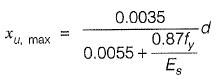for fy = 415 N/mm2
and Es = 2 x 105 N/mm2
xu, max = 0.48 d and
for  fy = 250 N/mm2
xu, max = 0.53 d

QUESTION: 3

### A reinforced concrete beam is subjected to the following bending moments: Dead load - 20 kN-m Live load - 30 kN-m Seismic load - 10 kN-m The design bending moment for limit state of collapse is

Solution:

The various load combinations are as follows:
M= 1.5 (DL + LL)
= 1.5 x (20 + 30) = 75 kN-m
Mu = 1.5 (DL + EL)
= 1.5 x (20 + 10) = 45 kN-m
Mu = 1.2 (DL + LL + EL)
= 1.2 x (20 + 30 + 10) = 72 kN-m
So, design BM is maximum of all three combinations i.e. 75 kN-m.

QUESTION: 4

Doubly reinforced beams are recommended when

Solution:
QUESTION: 5

In a cantilever beam carrying gravity load, main reinforcement is provided

Solution:

In cantilever beam the top fibers above the neutral axis are subjected to tensile stresses. So main reinforcement is provided above the neutral axis.

QUESTION: 6

A doubly reinforced concrete beam has effective cover d' to the centre of compression reinforcement, ‘xu' is the depth of neutral axis,  and 'd' is the effective depth to the centre of tension reinforcement. What is the maximum . strain in concrete at the level of compression reinforcement?

Solution: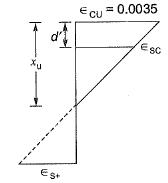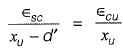∴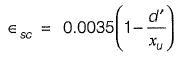QUESTION: 7

Consider the following statements:
In an under-reinforced concrete beam,
1. actual depth of neutral axis is less than the critical depth of neutral axis.
2. concrete reaches ultimate stress prior to steel reaching the ultimate stress.
3. moment of resistance is less than that of balanced sections.
4. lever arm of resisting couple is less than of balanced sections.
Which of these statements is/are correct?

Solution:

For under-reinforced beam,
(i) xu < xu, lim or xc
(ii) Steel reaches ultimate stress before concrete reaching the ultimate stress
(iii) Mu < Mu, lim
(iv) Lever arm = d - 0.42 xu will be more in the case of under-reinforced section.

QUESTION: 8

The maximum strain in the tension reinforcement in the section at failure when designed for the limit state of collapse should be
where, fy = Characteristic strength of steel, and Es = Modulus of elasticity of steel

Solution:

To ensure ductility the maximum strain in tension reinforcement in the section at failure shall not be less than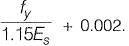QUESTION: 9

In the case of a continuous RC beam, in order to obtain the maximum positive span moment, where should the live load be placed?

Solution:
QUESTION: 10

Usually stiffness of a simply supported beam is satisfied if the ratio of its span to depth does not exceed which one of the following?

Solution:

For cantilever and continuous beam the ratio of span to depth to satisfy stiffness criterion shall not exceed 7 and 26 respectively.
(clause 23.2.1 of IS : 456-2000)

QUESTION: 11

In a singly reinforced concrete beam section, maximum compressive stress in concrete and tensile stress reach their permissible stresses simultaneously. What is such a section called?

Solution:
QUESTION: 12

What shall be the maximum area of reinforcement
(i) in compression and
(ii) intension to be provided in an RC beam, respectively, as per IS:456?

Solution:

As per clause 26.5.1.1 of IS : 456-2000, the maximum area of tension reinforcement in beams shall not exceed 0.04 bD.
As per clause 26.5.1.2 of IS : 456-2000, the maximum area of compression reinforcement in beams shall not exceed 0.04 bD.

QUESTION: 13

In limit state design method, the moment of resistance for a balanced section using M20 grade concrete and HYSD steel of grade Fe 415 is given by Mu.lim = Kbd2, what is the value of K?

Solution:

Moment of resistance for a balanced section is given by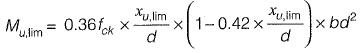But for Fe 415,
x u, lim = 0.48 d
∴ Mu.lim = 0.36 fck x 0.48 x (1 - 0.42 x 0.48) x bd2
⇒  Mu.lim = 0.13796 fck x bd2
On comparison, we get,
K = 0.13796 fck
= 0.13796 x 20 = 2.76

QUESTION: 14

How is the deflection in RC beams controlled as per IS:456?

Solution:
QUESTION: 15

What is the adoptable maximum spacing between vertical stirrups in an RCC beam of rectangular cross-section having an effective depth of 300 mm?

Solution:

As per clause 26.5.1.5 of IS: 456-2000, the maximum spacing of shear reinforcement measured along the axis of member shall not exceed 0.75 d for vertical stirrups and ‘d ’ for inclined stirrups at 45°, where 'd' is the effective depth of the section under consideration. In no case shall the spacing exceed 300 mm.
∴ Maximum spacing =0.75 d
= 0.75 x 300
= 225 mm

QUESTION: 16

A simply supported RC beam having clear span 5 m and support width 300 mm has the cross- section as shown in figure below.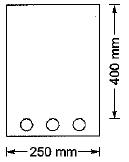What is the effective span of the beam as per 1S:456?

Solution:

As per clause 22.2 of IS:456-2000, for simply supported beam or slab, the effective span of a member that is not built integrally with its supports shall be taken as clear span plus the effective depth of slab or beam or centre to centre of supports, whichever is less.
∴ Effective span = 5 x 1000 + 400
= 5400 mm
and effective span =  5 x 1000 + (300/2) + (300/2)
= 5300 mm

QUESTION: 17

What is the modular ratio to be used in the analysis of RC beams using working stress method if the grade of concrete is M20?

Solution:

As per IS: 456-2000 modular ratio is given by,
m = (280/3σcbc)
For M20 concrete,
σcbc =  7N/mm2
∴ m = 280/(3x7) = 13.3

QUESTION: 18

In RCC beams, as the percentage area of tensile steel increases

Solution:
QUESTION: 19

The total compressive force at the time of failure of a concrete beam section of width 'b' without considering the partial safety factor of the material is
Where xu is the depth of neutral axis, fck cube strength of concrete.

Solution:

Total compressive force without considering the partial safety factor of material,
⇒ 0.36 fck b xx 1.5 = 0.54 fck b xu

QUESTION: 20

A floor slab of thickness t, is cast monolithically transverse to a rectangular continuous beam of span, L and width, B. If the distance between two consecutive points of contraflexure is, Lo, the effective width of compression flange at a continuous support is

Solution:

For T-beams effective width of compression flange,
⇒ bf = (L0/6) + b + 6t
For L-beam,
⇒ bf = (L0/12) + bw + 3D